# Grouping of the cell's

## Grouping of the cell's

• A limited amount of current can be drawn from a single cell or battery
• There are situations where single cell fails to meet the current requirement in a circuits
• To overcome the problem cells can be grouped in series and in parallel combinations or mixed grouping of cells is done in order to obtain a large value
of electric current

### (A) Series combination

• Figure below shows the two cells of emfs E1 and E2 and internal resistance r1 and r2 respectively connected in series combination through external resistance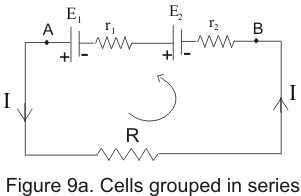• Points A and B in the circuit acts as two terminals of the combination
• Applying Kirchhoff's loop rule to above closed circuit
-Ir2-Ir1-IR+E1+E2=0
or
I=E1+E2/R+(r1+r2)
Where I is the current flowing through the external resistance R
• Let total internal resistance of the combination by r=r1+r2 and also let E=E1+E2 is the total EMF of the two cells
• Thus this combination of two cells acts as a cell of emf E=E1+E2 having total internal resistance r=r1+r2 as shown above in the figure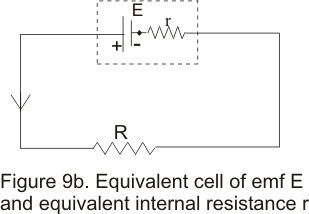### (B) Parallel combinations of cells

• Figure below shows the two cells of emf E1 and E2 and internal resistance r1 and r2 respectively connected in parallel combination through external resistance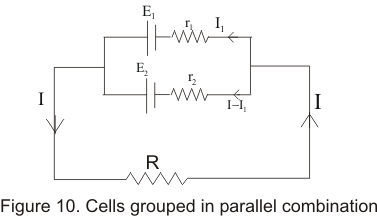• Applying Kirchhoff's loop rule in loop containing E1 ,r1 and R,we find
E1-IR-I1r1=0 ------------------------(1)
Similarly applying Kirchhoff's loop rule in loop containing E2 ,r2 and R,we find
E2-IR-(I-I1)r2=0 ------------------------(2)
• Now we have to solve equation 1 and 2 for the value of I,So multiplying 1 by r2 and 2 by r1 and then adding these equations results in following equation
IR(r1+r2)+r2r1I-E1r2-E2r1=0
which gives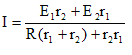We can rewrite this as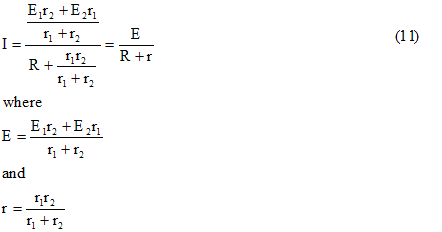E is the resulting EMF due to parallel combination of cells and r is resulting internal resistance.